# Writing a simple Compiler on my own - Creating a grammar for our Language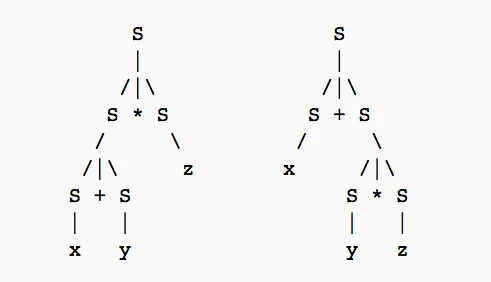Hello it's a me again Drifter Programming! Today we get to my Compiler series again to create a grammar for our Language! In my post "A simple C Language" I covered most stuff that has to do with our language which are all the tokens (keywords, operators, identifiers etc.) and the basic structure of such a "C-like" program, but we didn't create a grammar. This is exactly what we will do today!

So, without further do, let's get started!

## How we will write the grammar

Creating a grammar for a language directly in Bison rules is difficult and so I will try making it simpler to understand by writing some statements (for, while, definitions etc.) in the Language that we want to end up with.

This actually means that we will see where and how each token (keywords, operators etc.) is being used. That way we find out how the grammar and language is build up (in tokens) and can then define simple rules to form our first Bison parser!

You can clearly see that this method will make it much easier for us to write the grammar, because it will give us the fundamental building blocks of the language. After creating the basic structure we can of course insert more rules that will extend our grammar and we can even define more tokens (lexical units) that will be used in new statements.

## The Language

In the post "A simple C Language" we defined a simpler C-Language that is much simpler then the actual C Language and contains less tokens and statements.

Let's get into some of the main and fundamental things that the language contains.

In this language we can declare variables, arrays, pointers, functions of the types:

• char    (CHAR keyword)
• int    (INT keyword)
• float    (FLOAT keyword)
• double    (DOUBLE keyword)
• void    (VOID keyword)

We have conditional statements that use the keywords:

• IF
• ELSE
• ELSE IF     (combination)

We have loops that are made using the keywords:

• FOR    (for loop)
• WHILE    (while loop)

We have some other keywords that are for specific actions:

• CONTINUE -> go to the next iteration of a loop
• BREAK -> break out of a loop
• RETURN -> return to function that called (maybe even with return-value) or to the operating system

We have the following arithmetic and boolean operators:

• + and -    (addition and substraction operators)
• * and /    (multiplication and division)
• ++ and --    (increment and decrement)
• ||    (boolean or)
• !    (boolean not)
• &&    (boolean and)
•  == and !=    (equality and inequality)
•  > or < or >= or <=    (relational operators)

We also have:

• identifiers (ID)
• integer constants (ICONST)
• floating point constants(FCONST)
• printable ASCII characters (CCONST)
• sequences of characters (STRING)

Other tokens:

• '('   ')' -> parentheses
• '['   ']' -> brackets
• '{'   '}' -> bracelets
• ';'   '.'   ',' -> semi, dot and comma
• '='   '&' -> assign and reference
• (EOF) -> end of file
Directly from the previous post

And of course there are also comments of the forms:

• single-line comment (anything after "//")
• multiple-line comment (anything between "/*" and "*/")

All of that was already discussed in the post "A simple C language"!

But, how is the syntax of all those statements and operations?

Let's get started with the most simple one which is the definition of variables, arrays etc.

## Declarations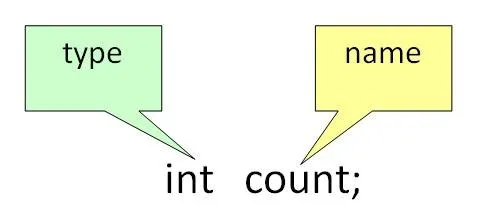To declare a variable we write something in the form:

``type name;``

The type will of course be anything from the types: int, char, float, double or void.

This means that we need to have a rule "type" (non-terminal) that has 5 right-sides which will be the type-keywords (terminals) for each of those 5 types.

The name on the other hand is an identifier (ID) and so a terminal symbol. We don't need to define a special rule for one variable, but we will have to when we get into more variables.

So, the variable declaration looks like this:

``declaration: type ID ';' ;``
``type: INT | CHAR | FLOAT | DOUBLE | VOID;``

For example:

float a;

Which is an integer variable with name/identifier a.

But this is not the final form...

Well, for one variable it's ok, but for more we will have to make a recursive rule which will allow more then one variable names (ID's), separated with comma (',').

So, the name-rule will be:

``names: ID | names ',' ID;``

This means that we have the following for declarations:

``declaration: type names ';' ;``
``type: INT | CHAR | FLOAT | DOUBLE | VOID;``
``names: ID | names ',' ID;``

But, what about arrays and pointers?

To make it easier for us we will define a rule for the variable that will replace ID.

So, a variable can be:

• ID     (simple variable)
• *ID, **D, *...*ID    (single, double, etc. pointer)
• ID[], ID[][], ID[]...[]    (1-, 2-, etc. dimensional array)

This means that the rule for a variable is:

``variable: ID | ('*')+ID | ID('['ICONST']')+;``

where the + is representing one or more occurences of the ().

You can also see that the dimension of an array is defined using an integer (ICONST)

So, the final declaration rule now is:

``declaration: type names ';' ;``
``type: INT | CHAR | FLOAT | DOUBLE | VOID;``
``names: variable | names ',' variable;``
``variable: ID | ('*')+ID | ID('['ICONST']')+;``

For example we could write:

char c, **str, A;

The last and final step is to put this rule in a recursion so that we can declare multiple variables, but all have to occur in the beginning of the main function or any other "user-declared" function. This makes it easier for us, cause it will not be possible to declare variables afterwards which makes the memory-allocation process much faster!

This looks like this:

``declarations: declarations declaration | declaration;``

## Conditional statement (if-else)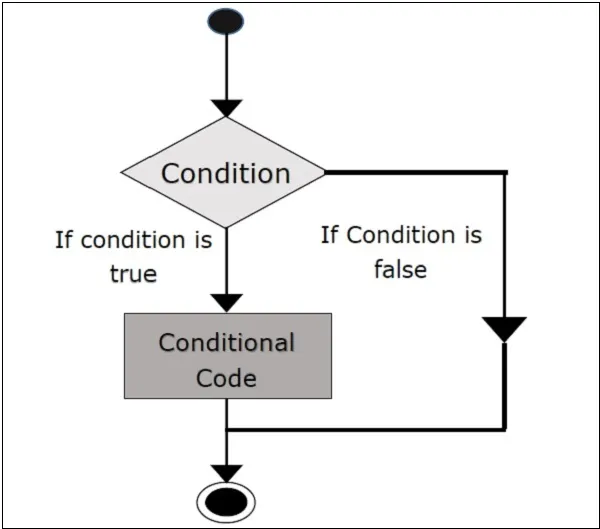We all know conditional statements.

For example we write:

``if(x == 0){``
``   // some action``
``}``
``else if(x == 1){``
``    // some action``
``}``
``else{``
``    // default action``
``}``

The "if" part is important and necessary, but the "else if" and "else" parts are not.

Also, we can have multiple "else if" parts, but only one "else" part!

This clearly shows us that we have the following structure:

``IF '(' bool_expression ')' '{' statements '}'``
``(ELSE IF '(' bool_expression ')' '{'statements '}')*``
``(ELSE '{' statements '}')?``

Where the * means zero or more times and ? means 0 or 1 times the ().

You can clearly see that we need to "define" what a "bool_expression" is and also what "statements" are. A "bool_expression" is a boolean expression which means that it gives us a value of true(1) or false(0) depending on the output of the condition it represents. "Statements" will be any type of expression, statement etc. that we can write inside of the "main body" of a program, function or if, while, for statement.

The {} part is not used when having only one statement and so we can also define "if" like that:

``IF '(' bool_expression ')' statement``

The same for "else if" and "else"...

So, to include this form also we can define something like a "tail" which will represent one statement or many statements inside of {}.

A tail-rule looks like this:

``tail: statement | '{' statements '}' ;``

And so the final if-rule is now:

``if_statement:``
``IF '(' bool_expression ')' tail``
``(ELSE IF '(' bool_expression ')' tail)*``
``(ELSE tail)?``
``;``

Where:

`` statements: statements statement | statement;``

statement and bool_expression (or simply expression) will be defined later...

## Loop statements (for and while)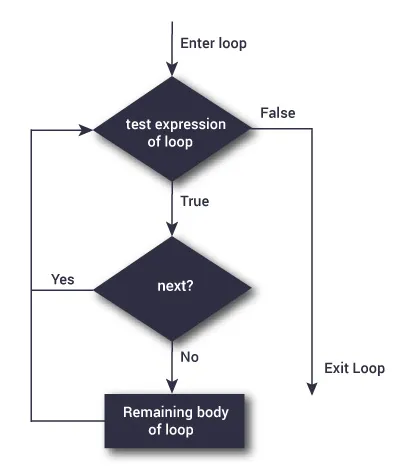Loop statements are also familiar...

Of course a for loop looks like this:

``for(expression ';' expression ';' expression){``
``    // action``
``}``

We can again have only one statement (like before), but the main part is the parenthesis that contains 3 expressions separated by 2 semicolons.

So, the rule of course looks like this:

``for_statement: FOR '(' expression ';' expression ';' expression ')' tail ;``

expression can be anything from boolean condition to assignment...

A while loop on the other hand looks like this:

``while(bool_expression){``
``    // action ``
``}``

This makes it even easier, cause it's the same as the first part of an if-statement!

So, the rule for while is:

``while_statement: WHILE '(' bool_expression ')' tail ;``

where bool_expression (as told before) will be an expression, but is a bool_expression for now so that we know the semantics. This means that the expression must be of "boolean type" and return a true-false value from an condition!

## Expressions

So, what are expressions?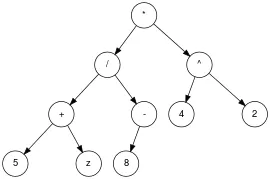Expressions are arithmetic or boolean operations between variables, constants and even other expressions! This means that we now have to define a rule for constants and all the arithmetic and boolean expressions.

The rule for a constant is:

``constant: ICONST | FCONST | CCONST ; ``

An expression is every possible operation, relation etc. and so:

``expression:``
``expression '+' expression |``
``expression '-' expression |``
``expression '*' expression |``
``expression '/' expression |``
``expression '++' |``
``expression '--' |``
``'++' expression |``
``'--' expression |``
``expression '||' expression |``
``expression '&&' expression |``
``'!' expression |``
``expression '==' expression |``
``expression '!=' expression |``
``expression '>' expression |``
``expression '<' expression |``
``expression '>=' expression |``
``expression '<=' expression |``
``'(' expression ')' |``
``variable |``
``'-'? constant``
``;``

We can clearly see that those expressions are forming a tree and the priorities and associativities of this grammar (how the tree will be formed) are not defined. This means that this part of our grammar is ambiguous!

## AssigmentsThe last important "statement" that we use in our code is giving a value to a variable.

The assigment is of course of the form:

``variable = expression;``

Because of the way that we defined a variable and an expression we are actually almost done!

But, there is also something else.

We might also want to set up a reference ('&') to a variable.

This means that we will include: '&' variable.

So, an assigment will be:

``assigment: '&'? variable '=' expression ';' ; ``

One thing worth mentioning is that we will not assign a string directly, cause it takes a lot of time and so the STRING will only be used in our custom compiler method print() that we might also put as an Keyword later on if we find difficulties implementing it in another way!

## Statements

What are statements?

Well, statements is anything that we do inside of the main-body of our program!

This contains:

• conditional statements (if)
• loop statements (for and while)
• assignments
• other keywords (continue, break, return)
• function call (will be implemented later on)
• print() ??? [if it becomes difficult to implement it in another way]

So, a statement is:

``statement:``
``if_statement | for_statement | while_statement | assigment |``
``CONTINUE | BREAK | RETURN``
``;``

Many statements are:

`` statements: statements statement | statement;``

We left out the function/subprogram stuff which includes:

• function declarations AND
• function calling

I will do it later on when we are sure that the compiler works right!

## Sum-up

So, let's sum up what we created till now and write our Bison code!

A program of our language has the structure:

``declarations``
``statements``
``functions (not implemented yet.``

So, our program-rule (for now) is:

``program: declarations statements ;``

And we implemented each other rule previously explaining how we get there...

## Bison grammar:

Lastly, what follows is our grammar code in Bison (only the rules) and I don't guarantee that they will work directly. I just hope that these rules will help you understand the grammar better!

Of course the grammar is ambiguous (easy to see in the expressions part, as we already mentioned there), but it is easy to get over it by using priorities and associativities in Bison (for semantics), something that we will do next time maybe!

So, our final rules in Bison are:

``program: declarations statements ;``
``declarations: declarations declaration | declaration;``
``declaration: type names ';' ;``
``type: INT | CHAR | FLOAT | DOUBLE | VOID;``
``names: variable | names ',' variable;``
``variable: ID |``
``    ('*')+ID |``
``    ID('['ICONST']')+``
``;``
``statements: statements statement | statement;``
``statement:``
``if_statement | for_statement | while_statement | assigment |``
``CONTINUE | BREAK | RETURN``
``;``
``if_statement:``
``IF '(' expression ')' tail``
``(ELSE IF '(' expression ')' tail)*``
``(ELSE tail)?``
``;``
``for_statement: FOR '(' expression ';' expression ';' expression ')' tail ;``
``while_statement: WHILE '(' bool_expression ')' tail ;``
``tail: statement | '{' statements '}' ;``
``expression:``
``    expression '+' expression |``
``    expression '-' expression |``
``    expression '*' expression |``
``    expression '/' expression |``
``    expression '++' |``
``    expression '--' |``
``    '++' expression |``
``    '--' expression |``
``    expression '||' expression |``
``    expression '&&' expression |``
``    '!' expression |``
``    expression '==' expression |``
``    expression '!=' expression |``
``    expression '>' expression |``
``    expression '<' expression |``
``    expression '>=' expression |``
``    expression '<=' expression |``
``    '(' expression ')' |``
``    variable |``
``    '-'? constant``
``;``
``constant: ICONST | FCONST | CCONST ;``
``assigment: '&'? variable '=' expression ';' ; ``

The ?, * and + parts are of course hypothetical and will be made into new recursive and non-recursive rules! We will take a look at that next time!

### Image Sources:### Previous posts of the series:

Introduction -> What is a compiler, what you have to know and what you will learn.

A simple C Language -> Simplified C, comparison with C, tokens, basic structure

Lexical Analysis using Flex -> Theory, Regular Expressions, Flex, Lexer

Symbol Table Structure -> Why Symbol Tables and Basic implementation

Using Symbol Table in the Lexer -> Flex and Symbol Table combination

Syntax Analysis Theory -> Syntax Analysis, grammar types and parsing

Bison basics -> Bison tutorial

And this is actually it and I hope that you enjoyed it!

Next time I'm thinking of combing Flex and Bison together, so that the Lexer "sends" the tokens to the Parser! We will also put some things into the Symbol Table!

Bye!

H2
H3
H4
3 columns
2 columns
1 column
Join the conversation now